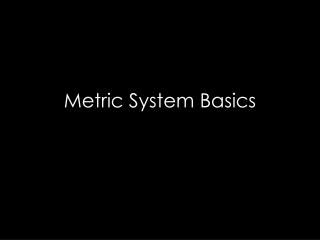DownloadDownload PresentationMetric System Basics

# Metric System Basics

Télécharger la présentation## Metric System Basics

- - - - - - - - - - - - - - - - - - - - - - - - - - - E N D - - - - - - - - - - - - - - - - - - - - - - - - - - -
##### Presentation Transcript

1. Metric System Basics

2. Metric System • The metric system is based on a base unit that corresponds to a certain kind of measurement • Length = meter • Volume = liter • Weight (Mass) = gram • Prefixes plus base units make up the metric system • Example: • Centi + meter = Centimeter • Kilo + liter = Kiloliter

3. Metric System • The three prefixes that we will use the most are: • kilo • centi • milli

4. Metric System • So if you needed to measure length you would choose meter as your base unit • Length of a tree branch • 1.5 meters • Length of a room • 5 meters • Length of a ball of twine stretched out • 25 meters

5. Metric System • But what if you need to measure a longer distance, like from your house to school? • Let’s say you live approximately 10 miles from school • 10 miles = 16093 meters • 16093 is a big number, but what if you could add a prefix onto the base unit to make it easier to manage: • 16093 meters = 16.093 kilometers (or 16.1 if rounded to 1 decimal place)

6. Metric System • These prefixes are based on powers of 10. What does this mean? • From each prefix every “step” is either: • 10 times larger or • 10 times smaller • For example • Centimeters are 10 times larger than millimeters • 1 centimeter = 10 millimeters

7. Metric System • Centimeters are 10 times larger than millimeters so it takes more millimeters for the same length 1 centimeter = 10 millimeters Example not to scale 40 41 40 41 1 cm

8. Metric System • For each “step” to right, you are multiplying by 10 • For example, let’s go from a base unit to centi 1 liter = 10 deciliters = 100 centiliters 2 grams = 20 decigrams = 200 centigrams ( 1 x 10 = 10) = (10 x 10 = 100) (2 x 10 = 20) = (20 x 10 = 200)

9. Metric System • An easy way to move within the metric system is by moving the decimal point one place for each “step” desired Example: change meters to centimeters 1 meter = 10 decimeters = 100 centimeters or 1.00 meter = 10.0 decimeters = 100. centimeters

10. Metric System • Now let’s try our previous example from meters to kilometers: 16093 meters = 1609.3 decameters = 160.93 hectometers = 16.093 kilometers • So for every “step” from the base unit to kilo, we moved the decimal 1 place to the left (the same direction as in the diagram below)

11. Metric System • If you move to the left in the diagram, move the decimal to the left • If you move to the right in the diagram, move the decimal to the right

12. Metric System • Now let’s start from centimeters and convert to kilometers 400000 centimeters = 4 kilometers 400000 centimeters = 4.00000 kilometers

13. Metric System • Now let’s start from meters and convert to kilometers 4000 meters = 4 kilometers • Now let’s start from centimeters and convert to meters • 4000 centimeters = 40 meters

14. Metric System • Now let’s start from meters and convert to centimeters 5 meters = 500 centimeters • Now let’s start from kilometers and convert to meters • .3 kilometers = 300 meters

15. Metric System • Now let’s start from kilometers and convert to millimeters 4 kilometers = 4000000 millimeters or 4 kilometers = 40 hectometers = 400 decameters = 4000 meters = 40000 decimeters = 400000 centimeters = 4000000 millimeters

16. Metric System • Summary • Base units in the metric system are meter, liter, gram • Metric system is based on powers of 10 • For conversions within the metric system, each “step” is 1 decimal place to the right or left • Using the diagram below, converting to the right, moves the decimal to the right and vice versa